Courses
Courses for Kids
Free study material
Free LIVE classes
More

# Center of a CircleLIVE
Join Vedantu’s FREE Mastercalss

## Introduction to Center of a Circle

A circle, in particular, is a simple closed curve that splits the plane into two areas: interior and exterior. In common usage, the term "circle" can refer either to the figure's boundary or the entire figure, including its interior; in strict technical everyday language, the circle is only the border, and the entire figure is called a disc. The center of a circle is the point where all the lines that form the boundary meet. The origin of circle is a point from which all distances to it are measured. In this article, we will be exploring origin of circle and its implications.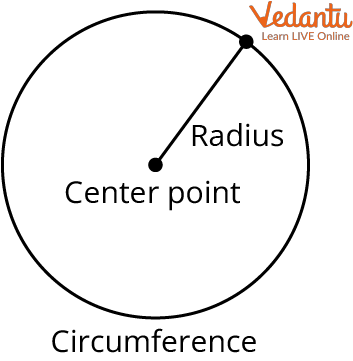Center of a Circle

## What is the Center of a Circle?

The point inside a circle that is equally spaced from all other points on the circle is considered the center of a circle.

It is a point inside the circle which is at an equal distance from all the points on its circumference.

The center of a circle is often referred to as the origin of a circle.

• A circle's radius-The radius of a circle is the distance between the circle's center and any point on its circumference.

• It is usually represented by the letters 'R' or 'r'.

• A circle's diameter- The diameter is the length of the line passing through the center and touching two points on the circle's boundary.

• A Circle's Chord-A straight line joining two points on the circumference of the circle is the chord of the circle.

• The diameter of a circle is defined as the circle's longest chord.

## How to Find the Center of a Circle?

Following is the method to find the origin of a circle by drawing chords.

1. Draw a chord in a circle (ab)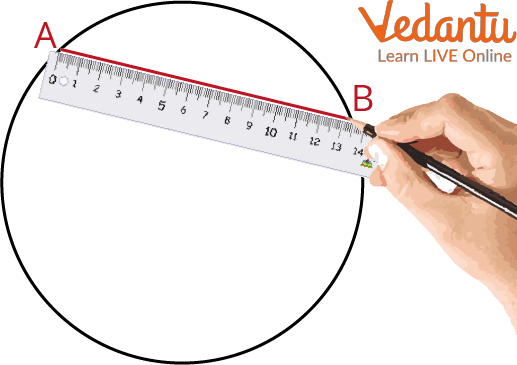Chord in a Circle

2. Draw another chord parallel to the previous chord (XY)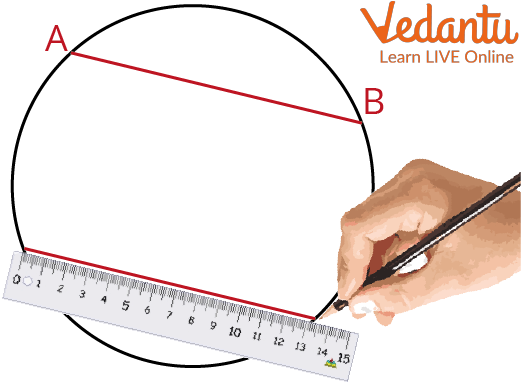Another Chord

3. Join points a and y Using a ruler

4. Join points b and x Using a ruler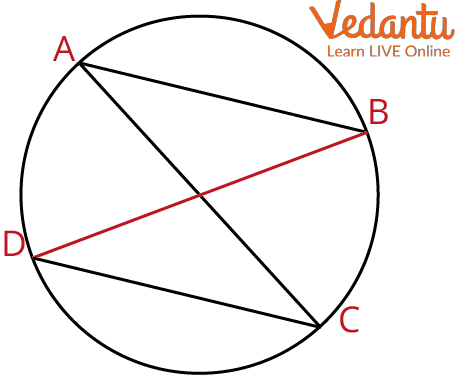Join the Points

5. The point where the lines intersect is the center of the circle.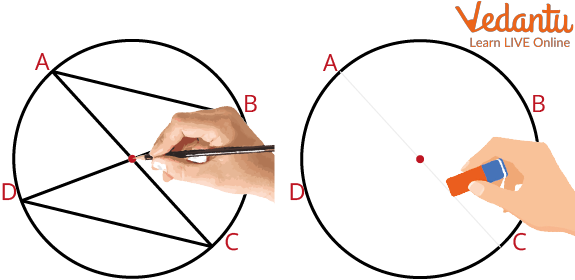The Lines Intersecting is the Center of the Circle

## Solved Example

Example 1: Find the area of a circle whose radius is 28 cm.

Ans: Given,

Radius of circular region = r = 28 cm

Area of a circle = $\pi\,r^2$

= $\dfrac{22}{7}\times 28 \times 28$

= $22\times 4\times21$

= 1848 sq. cm

Therefore, the area of the circular region is 1848 sq. cm.

Example 2: Tell whether the given statement is true or not: If the endpoints of the diameter of the circle are given, then to find the coordinates of the center we use the mid-point formula.

Ans: True, if the endpoints of the circle's diameter are given, then to find the coordinates of the center we use the mid-point formula.

## Practice Questions

Q1. Find the diameter of a circle whose radius is 10 cm.

Ans: 20cm

Q2. The radius of a circle is 2 cm. What is the diameter of a circle whose radius is 3 times that of the given circle?

Ans: 12cm

## Summary

So, from the article, we know that a circle is made up of all points in the same plane that are equidistant from one another. The center of a circle is the point equidistant from all points on the edge of the circle. It is also the point at which the circle's circumference (the line that defines the edge of the circle) intersects its diameter (the distance across the circle). The center of a circle is important in geometry because it is used to define many other geometric concepts, such as radius, chord, and arc.

Last updated date: 22nd Sep 2023
Total views: 114.3k
Views today: 4.14k

## FAQs on Center of a Circle

1. What is the circumference of a circle?

The circumference of a circle is its edge or the measurement of its entire arc. The circumference of a circle can be measured by opening it up and measuring its edge similarly to how we measure a straight line.

Even though a circle's circumference is equal to the length of its boundary, measuring it using a ruler or scale is not possible. Using the circle's radius (r) and the number "pi," one may calculate the circumference of a circle.

$2{\pi}r$ is used to find the circumference of a Circle.

2. How to calculate the radius of a circle?

The radius formula is quite easily obtained by halving the circle's diameter. The line segment created when we join a point on a circle's circumference to its exact center is referred to as the circle's radius. The radius of a circle is the separation between its center and circumference. Always, the diameter is twice the radius. As a result, the formula is created by multiplying the diameter by two.

Hence, Radius in Terms of Diameter is equal to $\dfrac{d}{2}$.

3. Name a few characteristics of a circle.

The following are some of the important characteristics of circles:

• A circle's circumference is equally spaced from its center.

• The circle is divided into two equal halves by its diameter.

• Equal-radius circles are equivalent to one another.

• Similarity exists between circles with various radii or sizes.

• The biggest chord in a circle is its diameter, which is twice as large as its radius.